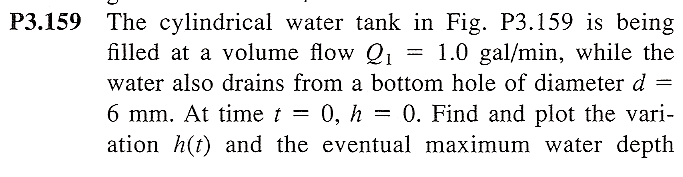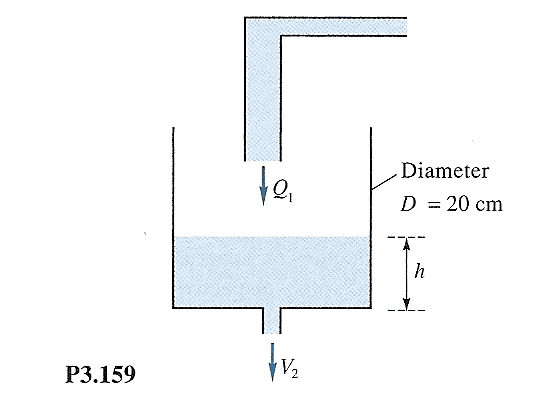# More Bernoulli finding h(t)

## Homework Statement## Homework Equations

Bernoulli eq and Conservation of Mass

## The Attempt at a Solution

Treating the ENTIRE system as my control volume, from conservation of mass we have:

$$\frac{d}{dt}\int\int\int_{cv}\rho\, dV +\dot{m_2}-\dot{m_1}=0$$

$$\Rightarrow \frac{d}{dt}\int_h\rho A_o\, dh+\rho v_2A_2-A_1Q_1=0$$

Now using Bernoulli, I will find V2.

My only question is this: I am going to apply the B.E. on a streamline that flows from the tank with diameter Do to the exit spout of diameter d. Am I correct in saying that Q1 does not affect this calculation of V2?

i.e. $v_2=\sqrt{2gh(t)}$

Looks like I will have a D.E. on my hands. Shouldn't be too bad though, just want to make sure that I am on the right track before continuing. This is what I have so far:

$$A\frac{dh}{dt}+B\sqrt{h}+C=0$$

where A=(pi/4)(Do2) B=(pi/4)(d2\sqrt(2g))

and C=-Q1

Hmm. Been awhile. This non-linear is it not? Is this one of those "Bernoulli eqs" ? Where I make it linear?

Last edited:
I'm not sure what your showing here. However, the problem looks like one of those flow rate problems that was in either late Calculus or early ODE's. Something along these lines:

The input rate Q1 * Delta T = Delta gallons. Translate this to Delta Volume = Fixed tank area * Delta H. Now subtract off the rate of the output. The output rate will probably be related to the pressure over the hole, where pressure is related to how much water is in the tank. This should lead to a D.E. that probably should raise to a certain height, exponentially, and then reach steady state.

For what it's worth, I did:

$$Q1 * \Delta T - Q2 * \Delta T = A_t * \Delta h$$

where $$A_t$$ is tank area and Q2 is the output rate.

A quick google search for fluid flow seems to indicate that Q2 = $$A_d * v_2$$ where $$A_d$$ is hole area and $$v_2$$ is what you gave in your post.

So dividing out by $$\Delta T$$ and taking the limit gives that same DE that you show above. Since this is first order, you could try to just integrate it by dividing the dh / (something in h) = constant * dt.

Of course I'm using gallons and volume loosly here. When numbers get plugged in the units have to be taken care of.

This is for a fluid mechanics class and is to be solved using Bernoulli equation. That is out I arrived at the D.E. I showed above.

Ah...never taken that as a course. The only other thing I can add is that my DE and thus yours is assuming that the water input is being added in an ideal fashion or "deposited" such that it doesn't directly affect the output rate. Thus, I now understand your original question of does a flow or current of some sort from the input affect the output? The picture has the input pipe directly over the output hole indicating that in a real situation a current of water will be happening that would affect the answer. Have you covered things like that in your class? I don't think enough information is given to take that into account (such has height of the upper pipe). So it looks like the problem was meant to be solved assuming the ideal case. Maybe someone smarter than I on fluid mechanics can add to this discussion.

No, I think you are correct Chrisas. We have yet to (and doubtfully will) cover the non-ideal case.

I guess you have already answered my real question. We have yet to come across a D.E. in this course (it's still early) and mt Professor has been pretty good at giving us everything we need to solve the HWs.

Thus I was wondering the whole time if I should be arriving at a D.E. But, you have answered that.

ThanksNow I just have to figure out the best way to solve that D.E. as it's been a good year and a half since that class ;)

you could try to just integrate it by dividing the dh / (something in h) = constant * dt.

Consider trying the above. I used my calculator to do the integration of the "h" side. The answer is two terms in "h" that probably could be gotten using integration by parts. The "t" side is simple. Notice that the problem says to graph the function to get the answer. This is because the answer can't be solved (at least by my calculator) in the form h = function of t. However, you do get t = function of h which can be plotted easily. Hopefully you will find that as t gets large, that h goes towards some constant value.

"plot the variation h(t)"

By the way, I think (and I need to go do this myself now to see) that you can use the DE to get the final answer without solving it. If h(t) goes to some constant value, then dh/dt goes to zero. So set dh/dt to zero in the DE and solve for h. That "should" match the value you get from the plot.

Can I just solve this:

$$A\frac{dh}{dt}+B\sqrt{h}+C=0$$

By dividing through by A and putting in the form:

$$\frac{dh}{dt}+\frac{B}{A}\sqrt{h}=-\frac{C}{A}$$

and use the integrating factor method?

Or does that sqrt mess it up?

Why not separate the variables and integrate?

$$\frac{A*dh}{C+B*\sqrt{h}}=-dt$$

A substitution of $$u=C+B*\sqrt{h}$$ makes the left side easy to integrate.